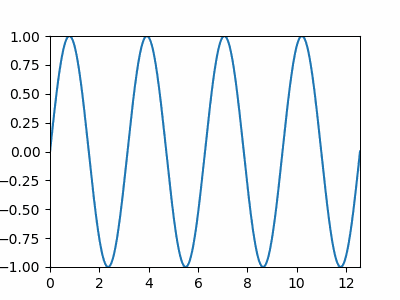# 如何使用 Matplotlib 實時繪製資料

## `FuncAnimation()` 函式

``````matplotlib.animation.FuncAnimation(fig,
func,
frames=None,
init_func=None,
fargs=None,
save_count=None,
*,
cache_frame_data=True,
**kwargs)
``````

``````import numpy as np
import matplotlib.pyplot as plt
from matplotlib.animation import FuncAnimation

x = []
y = []

figure, ax = plt.subplots(figsize=(4,3))
line, = ax.plot(x, y)
plt.axis([0, 4*np.pi, -1, 1])

def func_animate(i):
x = np.linspace(0, 4*np.pi, 1000)
y = np.sin(2 * (x - 0.1 * i))

line.set_data(x, y)

return line,

ani = FuncAnimation(figure,
func_animate,
frames=10,
interval=50)

ani.save(r'animation.gif', fps=10)

plt.show()

````````````ani = FuncAnimation(figure,
func_animate,
frames=10,
interval=50)
``````

`figure` 是圖形物件，其圖將被更新。

`func_animate` 是在每一幀都要呼叫的函式。它的第一個引數來自下一個值 `frames`

`frames = 10` 等於 `range(10)`。每幀將 0 到 9 的值傳遞給 `func_animate`。我們還可以為 interframe 分配一個幀，例如列表 `[0，1，3，7，12]`

``````ani.save('animation.gif', fps=10)
``````

## `canvas.draw()` 和 `canvas_flush_events()`

``````import numpy as np
import time
import matplotlib.pyplot as plt

x = np.linspace(0, 10, 100)
y = np.cos(x)

plt.ion()

figure, ax = plt.subplots(figsize=(8,6))
line1, = ax.plot(x, y)

plt.title("Dynamic Plot of sinx",fontsize=25)

plt.xlabel("X",fontsize=18)
plt.ylabel("sinX",fontsize=18)

for p in range(100):
updated_y = np.cos(x-0.05*p)

line1.set_xdata(x)
line1.set_ydata(updated_y)

figure.canvas.draw()

figure.canvas.flush_events()
time.sleep(0.1)

```````plt.ion()` 開啟互動模式。如果未呼叫該圖，則不會更新。

`canvas.flush_events()` 是一種基於 JavaScript 的方法，用於在每次迭代時清除圖形，以使後續圖形不會重疊。

## Matplotlib 實時散點圖

``````import numpy as np
import matplotlib.pyplot as plt
x=0
for i in range(100):
x=x+0.04
y = np.sin(x)
plt.scatter(x, y)
plt.title("Real Time plot")
plt.xlabel("x")
plt.ylabel("sinx")
plt.pause(0.05)

plt.show()
``````